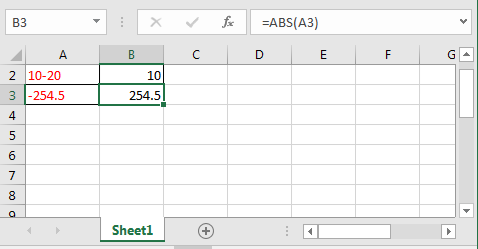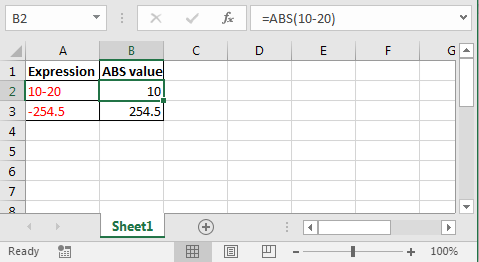# How to use the ABS function in ExcelIn this article, we will learn How to use the ABS function in Excel.

What is absolute value in mathematics ?

In Mathematics, there is an operator name absolute value. It only returns positive numbers but don't change the numerical value. For example absolute value of -12.5 be +12.5 and absolute value of +12.5 be +12.5

Excel provides a function to operate absolute value using the ABS function. For example, the square root of negative numbers is error in Excel. So use ABS function inside the square root operation in Excel. Or obtaining subtraction of two values and doesn't care about the sign of result. Let's learn ABS function syntax and an example to illustrate the function usage as shown below

ABS Function in Excel

The ABS function just changes the negative numbers to positive numbers but don't change the positive number

ABS Function syntax:

 =ABS(number)

number : number value

Example :

All of these might be confusing to understand. Let's understand how to use the function using an example. Here In Cell A2 I am subtracting 20 from 10. When I encapsulate it with the ABS formula, see what we get.

 =ABS(10-20)We get 10 instead of -10.

That’s it. This is how you get absolute value in Excel.

When and how to use ABS function?

You can use this function where values are always positive. Like distance.

Supply any expression to the ABS function, which will result in a number. ABS function will return the distance of that number from 0 on the number line. And this what absolute value means.

Here are all the observational notes using the ABS function in Excel
Notes :

1. The formula only works with numbers.
2. The formula returns the number rounded off to any decimal places.
3. Negative argument as decimal rounds the number to integer side.
4. Blank cells as an argument takes as zero value and returns 0 as result, so make sure to check blank cells before.
5. The function returns #VALUE! Error if the text value or numbers as text value.

Hope this article about How to use the ABS function in Excel is explanatory. Find more articles on calculating values and related Excel formulas here. If you liked our blogs, share it with your friends on Facebook. And also you can follow us on Twitter and Facebook. We would love to hear from you, do let us know how we can improve, complement or innovate our work and make it better for you. Write to us at info@exceltip.com.

Related Articles :

How to use the ROUND function in Excel | rounds off the given number to the nearest num_digit decimal using the ROUND function in Excel

How to use the ROUNDUP function in Excel | rounds up the given number to the nearest num_digit decimal using the ROUNDUP function in Excel

How to use the ROUNDDOWN function in Excel | rounds down the given number to the nearest num_digit decimal using the ROUNDDOWN function in Excel

How to use Excel F.TEST Function in Excel : The F.TEST Function is used to calculate F statistic of two samples in excel internally and returns the two tailed probability of the F statistic under Null Hypothesis.

How To Use Excel T.TEST Function in Excel : The T.TEST function is used to determine the confidence of an analysis in Excel. Mathematically, it is used to know if the mean of the two samples are equal or not. T.TEST function is used to accept or reject the null hypothesis.

How to use the DEVSQ Function in Excel : DEVSQ function is a built-in statistical function to calculate the sum of squared deviations from the mean or average of the given distribution provided.

How to calculate Standard Deviation in Excel : To calculate the standard deviation we have different functions in Excel. The standard deviation is the square root of the variance value but It tells more about the dataset than variance.

How to use the VAR function in Excel : Calculate the variance for the sample dataset in excel using the VAR function in Excel.

Popular Articles :

How to use the IF Function in Excel : The IF statement in Excel checks the condition and returns a specific value if the condition is TRUE or returns another specific value if FALSE.

How to use the VLOOKUP Function in Excel : This is one of the most used and popular functions of excel that is used to lookup value from different ranges and sheets.

How to use the SUMIF Function in Excel : This is another dashboard essential function. This helps you sum up values on specific conditions.

How to use the COUNTIF Function in Excel : Count values with conditions using this amazing function. You don't need to filter your data to count specific values. Countif function is essential to prepare your dashboard.

Terms and Conditions of use

The applications/code on this site are distributed as is and without warranties or liability. In no event shall the owner of the copyrights, or the authors of the applications/code be liable for any loss of profit, any problems or any damage resulting from the use or evaluation of the applications/code.